# Set Based Search Modeling Examples Andrew Kuipers amkuipercpsc

• Slides: 10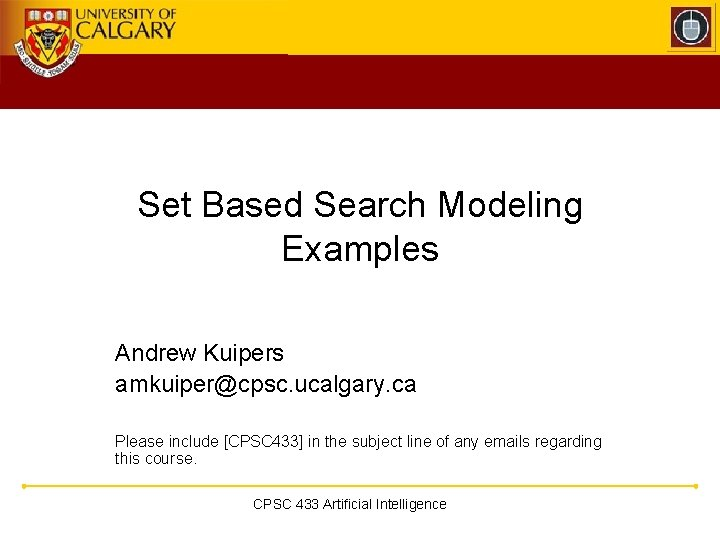Set Based Search Modeling Examples Andrew Kuipers [email protected] ucalgary. ca Please include [CPSC 433] in the subject line of any emails regarding this course. CPSC 433 Artificial Intelligence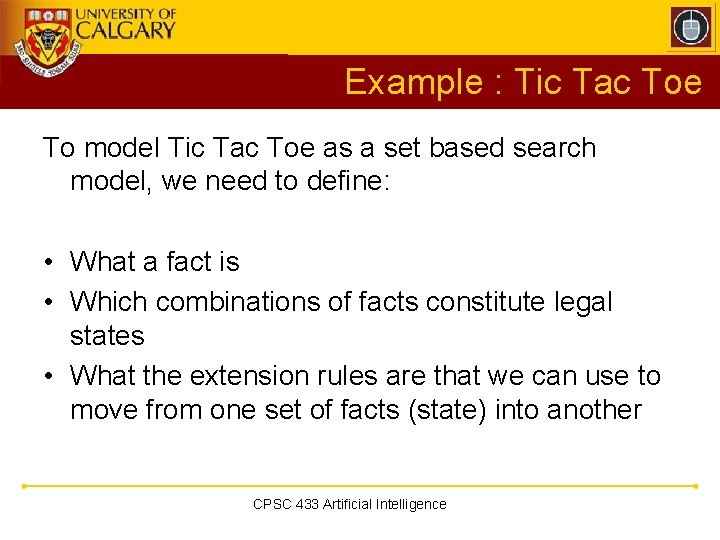Example : Tic Tac Toe To model Tic Tac Toe as a set based search model, we need to define: • What a fact is • Which combinations of facts constitute legal states • What the extension rules are that we can use to move from one set of facts (state) into another CPSC 433 Artificial IntelligenceExample : Tic Tac Toe Facts • A fact will simply be that a certain mark is made at a specific location on the tic-tac-toe board let R = {1, …, 3} let M = {X, O, B} (note: we’re also modeling blanks as marks) F = { (i, j, m) | i, j R, m M } CPSC 433 Artificial Intelligence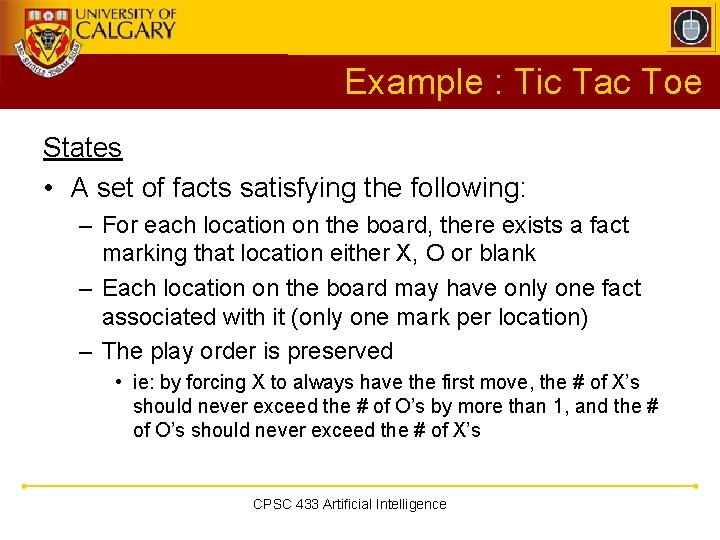Example : Tic Tac Toe States • A set of facts satisfying the following: – For each location on the board, there exists a fact marking that location either X, O or blank – Each location on the board may have only one fact associated with it (only one mark per location) – The play order is preserved • ie: by forcing X to always have the first move, the # of X’s should never exceed the # of O’s by more than 1, and the # of O’s should never exceed the # of X’s CPSC 433 Artificial IntelligenceExample : Tic Tac Toe States S = { F’ F | Covered(F’) All. Unique(F’) Play. Order. Preserved(F’) } Covered(F) i, j R m M | (i, j, m) F All. Unique(F) i, j R m, m’ M | ((i, j, m) F (i, j, m’) F) → (m = m’) CPSC 433 Artificial Intelligence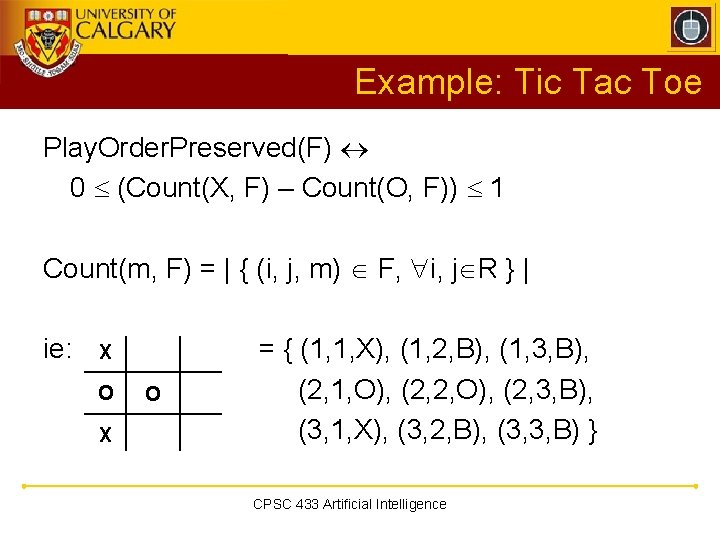Example: Tic Tac Toe Play. Order. Preserved(F) 0 (Count(X, F) – Count(O, F)) 1 Count(m, F) = | { (i, j, m) F, i, j R } | ie: X O = { (1, 1, X), (1, 2, B), (1, 3, B), (2, 1, O), (2, 2, O), (2, 3, B), (3, 1, X), (3, 2, B), (3, 3, B) } CPSC 433 Artificial Intelligence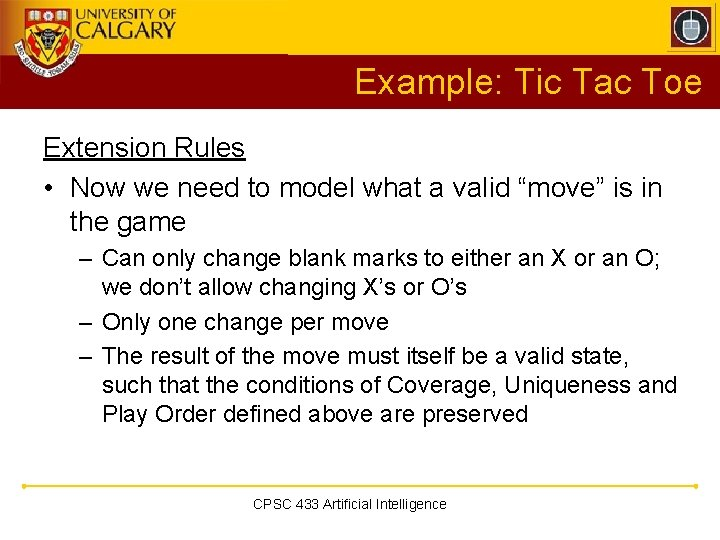Example: Tic Tac Toe Extension Rules • Now we need to model what a valid “move” is in the game – Can only change blank marks to either an X or an O; we don’t allow changing X’s or O’s – Only one change per move – The result of the move must itself be a valid state, such that the conditions of Coverage, Uniqueness and Play Order defined above are preserved CPSC 433 Artificial Intelligence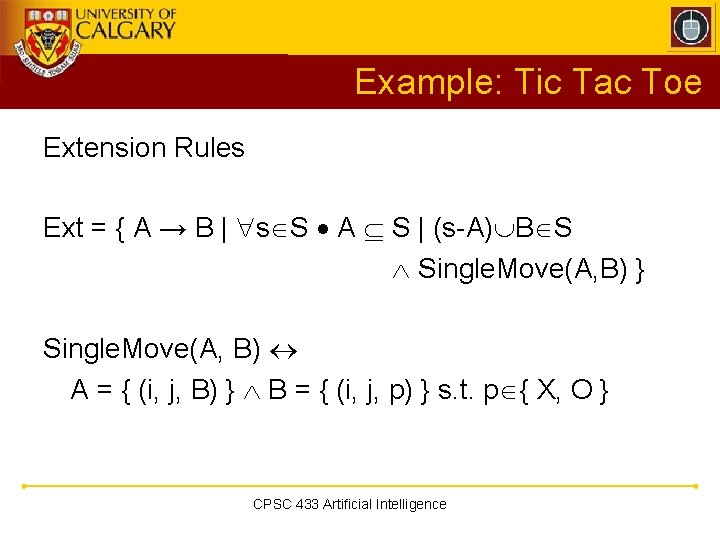Example: Tic Tac Toe Extension Rules Ext = { A → B | s S A S | (s-A) B S Single. Move(A, B) } Single. Move(A, B) A = { (i, j, B) } B = { (i, j, p) } s. t. p { X, O } CPSC 433 Artificial Intelligence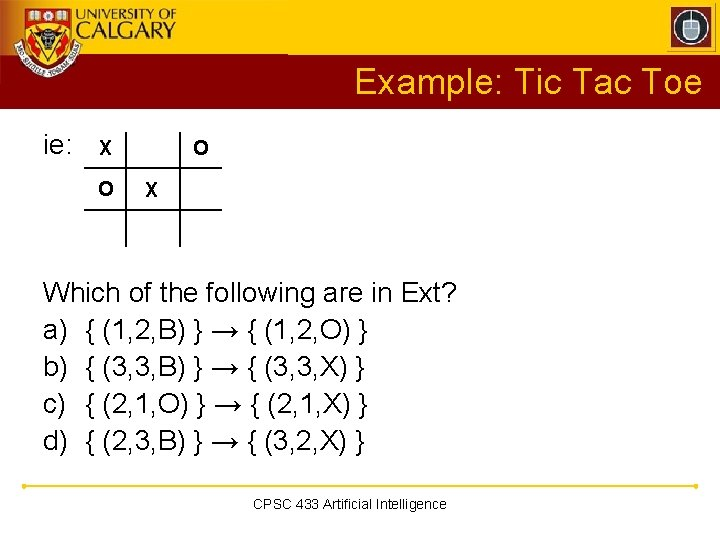Example: Tic Tac Toe ie: X O O X Which of the following are in Ext? a) { (1, 2, B) } → { (1, 2, O) } b) { (3, 3, B) } → { (3, 3, X) } c) { (2, 1, O) } → { (2, 1, X) } d) { (2, 3, B) } → { (3, 2, X) } CPSC 433 Artificial Intelligence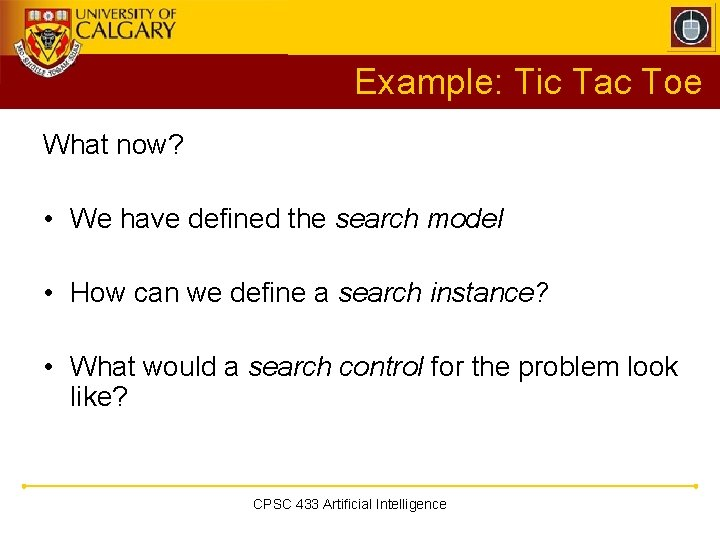Example: Tic Tac Toe What now? • We have defined the search model • How can we define a search instance? • What would a search control for the problem look like? CPSC 433 Artificial Intelligence